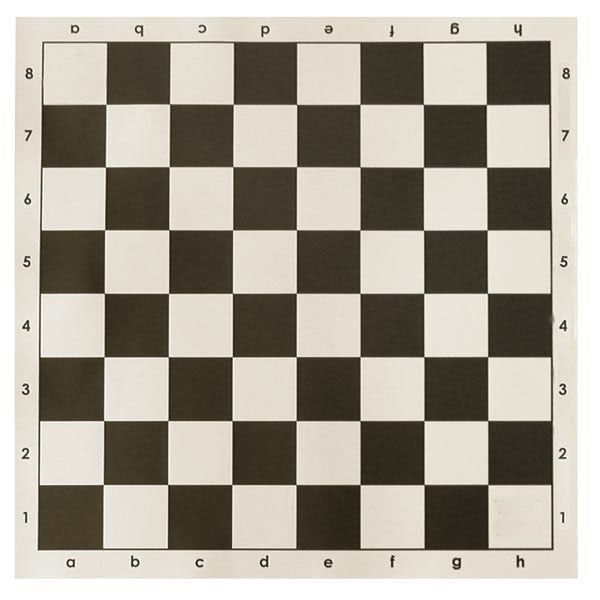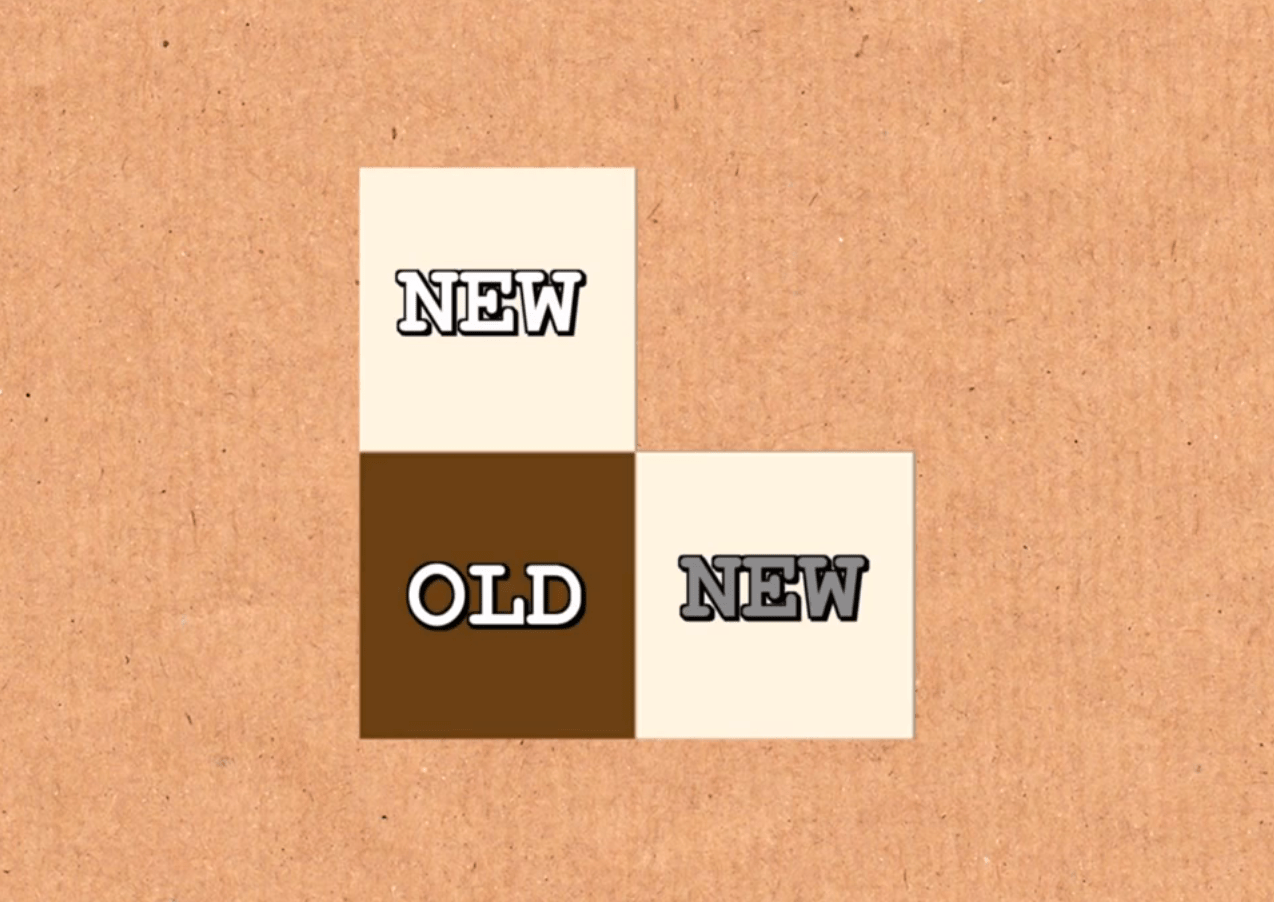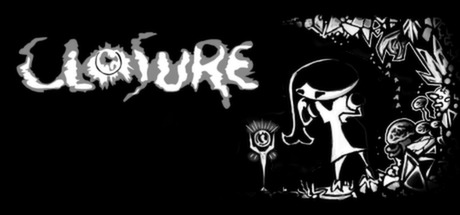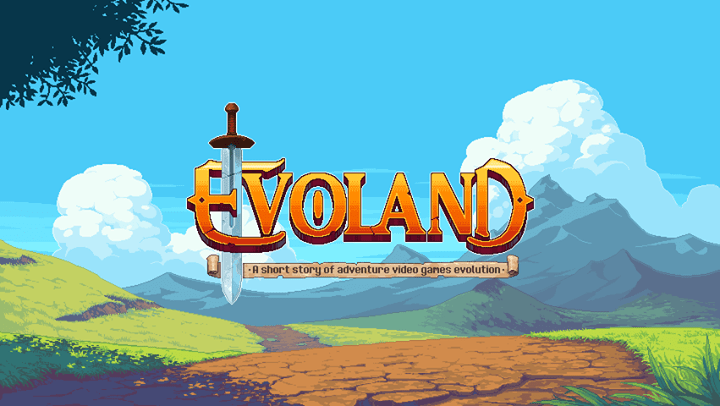# For the First Time in Forever

Frozen不是一个悲伤的电影，但却让我看到泪流满面。

# 棋盘上的越狱游戏.

.

.

.

.

.

.

.

.

.$$S=1+1/2+1/4+1/8+\dots$$

$$2S=2+1+1/2+1/4+1/8+\dots$$

$$2S=2+S$$

$$A=9/10+9/100+9/1000+9/10000+\dots$$$$9/10+9/100+9/1000+9/10000+\dots=0.9+0.09+0.009+0.0009+\dots=0.99999999999 \dots$$

$$0.99999999\dots=1$$

# 独立游戏推荐（3）

### Closure（Steam）### Evoland（Steam）# Spotify Plugin for Quicksilver

2014/1/5： 删除更改了一些多余/老旧的代码，没有新功能，用上去应该一样吧…

2013/11/2： 修正了一些小错误，添加了说明部分

# 《言の葉の庭》观感

======剧透部分到此为止======